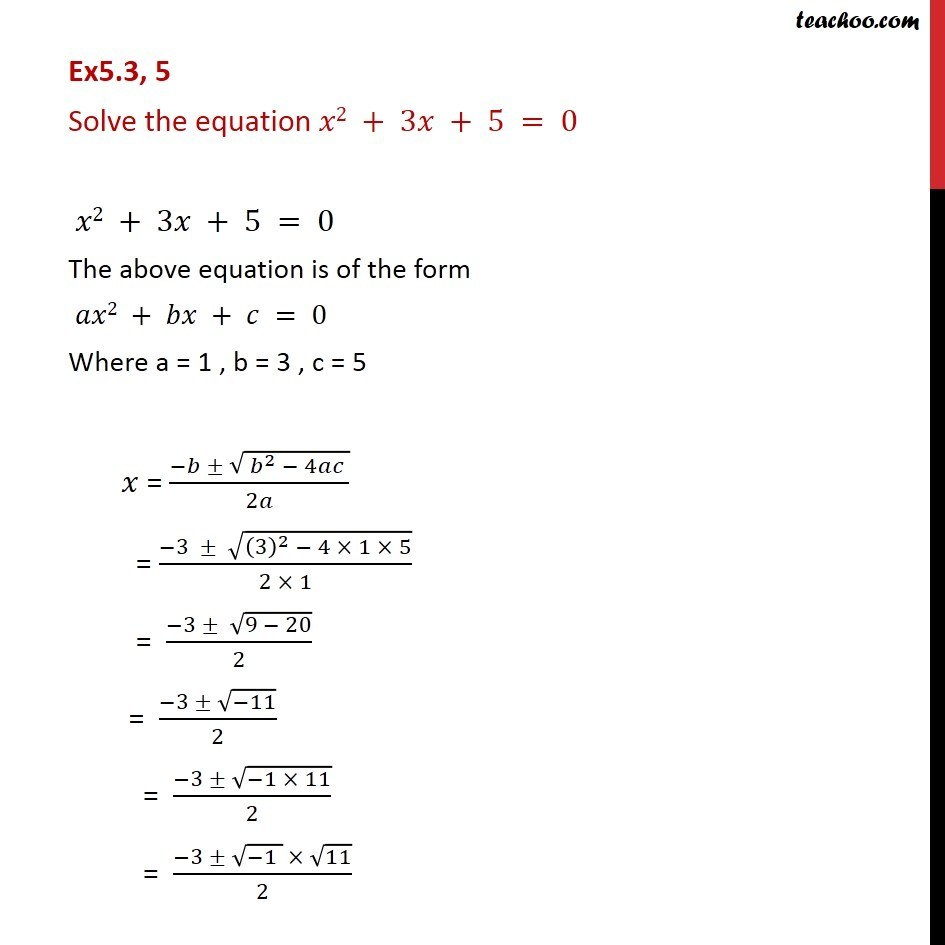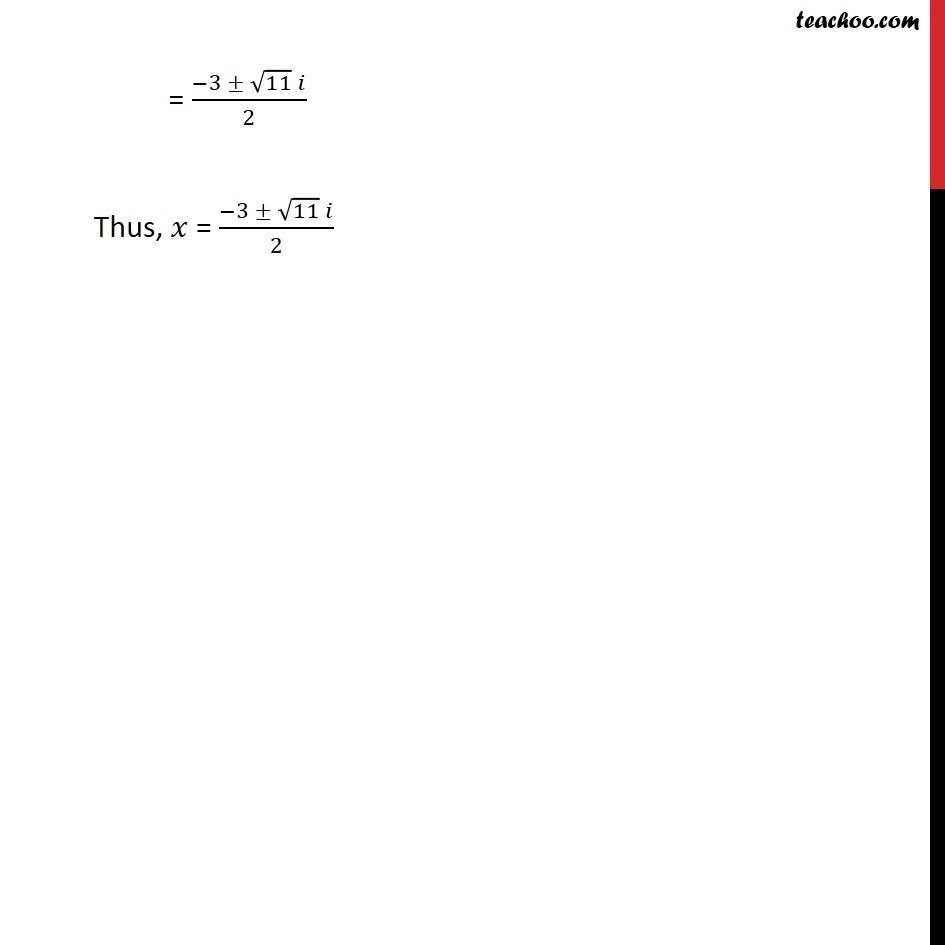Chapter 4 Class 11 Complex Numbers
Concept wiseLearn in your speed, with individual attention - Teachoo Maths 1-on-1 Class

### Transcript

Ex5.3, 5 Solve the equation 2 + 3 + 5 = 0 2 + 3 + 5 = 0 The above equation is of the form 2 + + = 0 Where a = 1 , b = 3 , c = 5 = ( ( ^2 4 ))/2 = ( 3 ((3)^2 4 1 5))/(2 1) = ( 3 (9 20))/2 = ( 3 ( 11))/2 = ( 3 ( 1 11))/2 = ( 3 ( 1 ) 11)/2 = ( 3 11 )/2 Thus, = ( 3 11 )/2